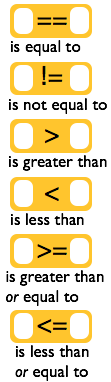# Booleans and Comparison Operators

## Word Soup: Conditionals, Booleans, expressions, statements

Historical Notes on Booleans
• Named after mathematician George Boole
• He invented a corner of mathematics that is now named after him called "Boolean Algebra"
• Boolean Algebra is math that operates using only true/false values.
• This is important work for computer science because true/false maps very easily to binary.
• As you saw in the video there are a bunch of terms that generally are all talking about the same thing.

Boolean, Boolean values, Boolean expressions:

• A Boolean value is simply a computer science-y term that means a true/false value.
• A Boolean expression is a statement that evaluates to a Boolean value (a single true/false).

Condition, Conditionals, Conditional Statements:

• "Conditional" is simply a generic term for code that alters program flow based on true/false values (like an `if` statement)
• Examples: Condition, Conditionals, Conditional statements, conditional execution

## Comparison OperatorsA common type of condition to check is a comparison of two values. Here are 6 common comparison operators. Each compares a value on the left with a value on the right and returns a Boolean value -- true or false. Most of these do what you would expect.

### Why these symbols: ==, !=, <=, and >=?

1. We use `==` because the single equal sign `=` is the assignment operator. We need something different to indicate we want to compare two values instead of assign one to the other.

Common mistake: writing something like `if (age = 18)` instead of `if (age == 18)`. We'll make sure we get this down later.

2. We use `!=`, `<=`, and `>=` because they only require ASCII symbols. Historically the mathematical symbols `≠`, `≤` and `≥` were hard or impossible to produce on some systems. The `!` is universally read as "not".

## Reference: Examples

Below are a bunch of examples of how you might see comparisons in code. Review them if you like or continue on and come back if you need reference.Compares two values - numbers, strings, or other booleans - and returns true if they are equal, otherwise false.

• `"Hello" == "hello"` returns false -- because the strings are are capitalized differently.
• `"3" == 3` returns true -- because `==` tries to be forgiving. If it can "coerce" a string into a number it will do so to compare. 1
• `(2+1) == 3` returns true -- because the arithmetic expression evaluates to 3.
• `x == 7` returns true -- when the variable x has the value 7.

1. While it is a useful feature that `==` will coerce a string into a number, it is considered TRICKY because the string "3" is not the same as the integer 3. There are times when you would believe these are not equal. There is a "strict" equality operator - the "triple equal" `===` which makes sure that both the type of data and value are equal. So ` "3" === 3 ` is false.Compares two values - numbers, strings, or other booleans - and returns `true` if they are not equal, otherwise `false`.

• `"Hello" != "hello"` returns true -- because the strings are slightly different.
• `"3" != 3` returns false -- because the string 3 can be coerced into a number before comparing with 3. (see notes above about the forgiving ==).
• `(2+1) != 3` returns false -- because the arithmetic expression evaluates to 3.
• `x != 7` returns true -- when the variable x is any value other than 7.Compares two values to see if the number on the left is greater than the number on the right.

• `4 > 3` returns true
• `3 > 7` returns false
• `age > 17` returns true -- when the value of the variable "age" is strictly greater than 17, otherwise false.Compares two values to see if the number on the left is less than the number on the right.

• `4 < 3` returns false
• `3 < 7` returns true
• `age < 17` returns true -- when the value of the variable "age" is strictly less than 17, otherwise false.Compares two values to see if the number on the left is less than or equal to the number on the right.

• `3 <= 4` returns true
• `4 <= 3` returns false
• `age <= 18` returns true -- when the value of the variable "age" is 18 or less.Compares two values to see if the number on the left is greater than or equal to the number on the right.

• `3 >= 4` returns false
• `4 >= 3` returns true
• `age >= 18` returns true -- when the value of the variable "age" is 18 or greater.

Found a bug in the documentation? Let us know at documentation@code.org